# Abstract

The reconstruction of the individual transmissions events that led to an outbreak gives valuable information on the causes of spread of an infectious disease. In recent years, methods have been developed to infer transmission trees from onset dates and genetic sequences. Nevertheless, sequences can be uninformative for pathogens with slow mutation rates, or sequencing can be scarce. We developed the package o2geosocial to integrate more variables from routinely collected surveillance data to reconstruct transmission trees when genetic sequences are not informative. The model introduced in o2geosocial takes the reported age-group, onset date, location and genotype of infected cases to infer probabilistic transmission trees. In this vignette, we describe the structure and the different functions of the package. We also provide a tutorial on a simulated measles outbreak showing how to run a model, evaluate the output and visualise the results of the inference. In the second part of the tutorial, we customise the likelihood functions to introduce a different mobility model.

# Introduction

The risk of outbreak in a given region is higher if there is low immunity against the virus in the population. Regional immunity against infectious diseases is built up by past infections and, if a vaccine is available, vaccination campaigns. Social and spatial heterogeneity in disease incidence or vaccine coverage lead to under-immunised areas, also called pockets of susceptibles. Importation of cases into these areas can cause large transmission clusters and long-lasting outbreaks. The most vulnerable areas of a country could be identified using historical data on local vaccine coverage and incidence, but these data are often scarce, or unreliable. Another solution is to infer probabilistic transmission trees and clusters to identify in which regions importations repeatedly caused large clusters of local transmission.

The Wallinga-Teunis method was developed to infer probabilistic transmission trees from onset dates, serial intervals and latent periods in a maximum likelihood framework. As genetic sequencing of pathogens during an outbreak became more common, new tools such as the R package outbreaker2 showed that combining the timing of infection and the genetic sequences could improve the accuracy of inferred transmission trees. Nevertheless, sequencing pathogens remains costly, and the efficacy of the reconstruction methods depends on the proportion of sequenced cases, the quality of the sequences, and on the characteristics of the pathogen. For instance, the measles virus evolves very slowly, so sequences from unrelated cases can be very similar, which makes methods combining onset dates and genetic sequences ineffective.

Building upon the framework presented in outbreaker2, we developed the R package o2geosocial to estimate the cluster size distribution from the onset date, age, location and genotype of the cases. Those variables are routinely collected by surveillance systems and often well reported. Using age-stratified contact matrices and mobility models, we combined the different variables into a likelihood of connection between cases. The package o2geosocial is ideal to study outbreaks where sequences are uninformative, either because only a small proportion of cases were sequenced or because the virus evolves too slowly. In this vignette, we first introduce the overall structure of o2geosocial, the components of the individual likelihood and the parameters; then we present a tutorial on how to develop a model to reconstruct the cluster size distribution with o2geosocial.

# Implementation

## Overall structure

The general implementation of o2geosocial follows the structure of outbreaker2 and incorporates C++ functions into a R framework using Rcpp. The main function of the package, called outbreaker(), uses Monte Carlo Markov Chains (MCMC) to sample from the posterior distribution. For each case, it infers the infection date, the infector, and the number of missing generations between the case and their infector. It takes five lists as inputs: i) ‘moves’, ii) ‘likelihoods’, iii) ‘priors’, iv) ‘data’, and v) ‘config’. These five lists can be generated and modified using the functions custom_moves(), custom_likelihoods(), custom_priors(), create_config() and outbreaker_data(). Examples of how these functions are used to customize the model will be presented in the Tutorial section.

The package o2geosocial was developed for the study of outbreaks where full information on the onset date, location and age group of the cases is available. It builds upon outbreaker2 by adding the effect of the location and the age-stratified contact data on the reconstruction of the transmission clusters. However, unlike outbreaker2, o2geosocial does not take genetic sequences as input. Genetic information (or genotype) is only used to exclude connections between cases, i.e. two cases cannot be from the same cluster if they belong to different genetic groups. Therefore, o2geosocial is adapted to reconstructing transmission clusters from large datasets (up to several thousand cases) where genetic sequencing is not informative, either because the mutation rate of the virus is slow, or because sequencing is scarce. On the other hand, outbreaker2 is more adapted to reconstructing transmission chains if genetic sequences are informative and available for a high proportion of the cases.

In o2geosocial the genetic component of the likelihood is a binary value depending on the genotype of the cases. There can be only one genotype reported per transmission tree, hence the number of trees will be at least equal to the number of unique genotypes reported in the dataset. The genotype of a tree T is the genotype reported for at least one of the cases belonging to T. For each genotyped case $$i_{gen}$$ and at every iteration, only cases from trees with the same genotype as $$i_{gen}$$, or without reported genotype should be listed as potential infectors. Therefore, ungenotyped cases belonging to a tree with a different genotype will not be potential infectors of $$i_{gen}$$ at this iteration. Ungenotyped cases can be placed on any tree.

Routinely collected surveillance data may include thousands of cases from unrelated outbreaks. Therefore, it was crucial to develop the algorithm to be computationally efficient. We added a pre-clustering step to the function outbreaker() to reduce the pool of potential infectors for each case, which can greatly reduce the computing time of the MCMC (Figure 1). In the pre-clustering step, the potential infectors for each case are listed. Cases with overlapping potential infectors, and their potential infectors, are grouped together. Cases from different groups cannot infect one another. The group each case belongs to is listed in the variable ‘is_cluster’, which is an element of the list returned by the function outbreaker_data(). We used three criteria to group together cases that may be connected: For each case $$i$$, only cases reported in a radius of $$\gamma$$ km, less than $$\delta$$ days before $$i$$, and from similar or unreported genotype can be classified as potential infectors of $$i$$. The parameter $$\gamma$$ and $$\delta$$ are defined as inputs in the function create_config().

Cases classified in the same group after the pre-clustering stage may still be unrelated (e.g. if several importations were reported simultaneously in a nearby region). Because of this, we defined a likelihood threshold $$\lambda$$ which allows connections to be discarded as unlikely. If the likelihood of connection between cases $$i$$ and $$j$$ is less than $$\lambda$$, we consider it to be more likely that $$i$$ was an import and unrelated to $$j$$. In o2geosocial, the variable $$\lambda$$ can either be an absolute (the log-likelihood threshold will be $$log(\lambda)$$) or relative value (a quantile of the likelihood of all connections in all samples), and is defined by the variables ‘outlier_threshold’ and ‘outlier_relative’ in create_config(). If the initial number of importations is too small, unlikely connections between cases can alter the inferred infection dates of cases and bias the generated transmission trees. Therefore, we first run a short MCMC and compute $$n$$, the minimum number of connections with a likelihood lower than $$\lambda$$ in the samples; then we add $$n$$ imports to the starting tree and run a long MCMC. Finally, we remove the connections with likelihood lower than $$\lambda$$ in the final samples and return the infector, infection date and probability of being an import for each case (Figure 1).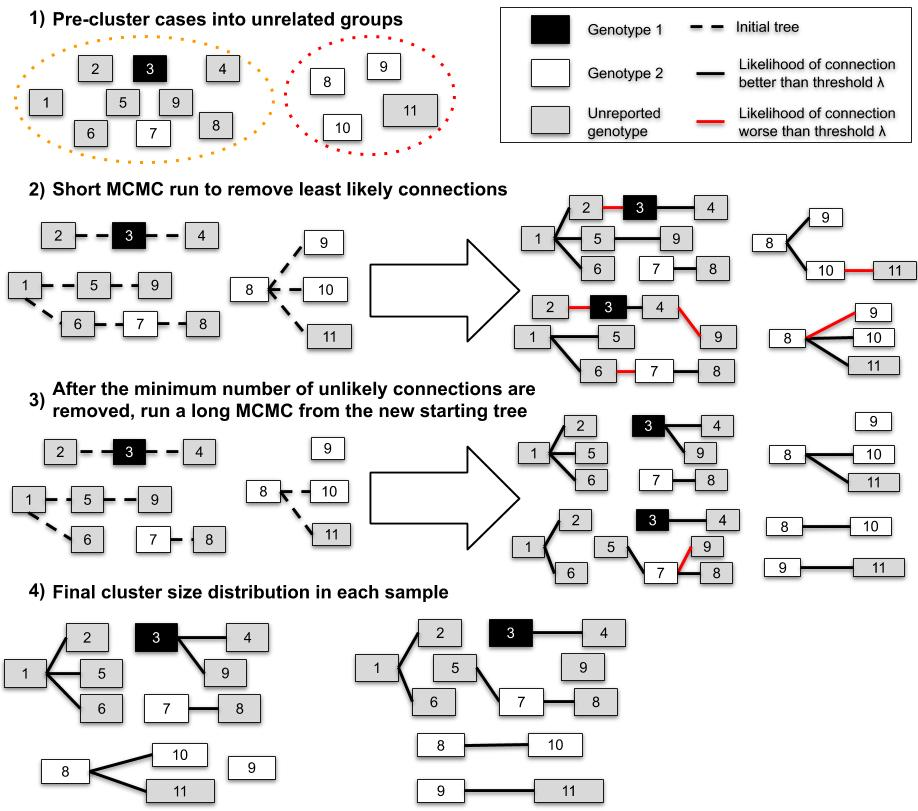Figure 1: Example of the process to estimate the cluster size distribution and the import status of $$11$$ cases. In the first step, cases are split in two groups that do not have overlapping potential infectors (they were reported in different places, or different time). In step 2, we estimate the minimum number of unlikely transmissions ($$n$$) in the samples (right panel). In step 3, we remove $$n$$ transmissions from the initial tree, and generate samples. Finally, we remove the unlikely connections in each sample of Step 3 and compute the inferred cluster size distribution.

## Likelihood and priors

The functions custom_likelihoods() and custom_priors() can be used to edit each component of the likelihood and priors.

### Genotype

The genetic component of the likelihood that a case $$i$$ of genotype $$g_i$$ was infected by a case $$j$$ belonging to the tree $$\tau_j$$ is defined as a binary value: $G(g_{i},g_{\tau_{j}})= \left\{\begin{array}{l} 1\text{ if }g_{i}\text{ unknown}\\ 1\text{ if }g_{\tau_{j}}\text{ unknown }\\ 1\text{ if }g_{i}\text{ and }g_{\tau_{j}}\text{ both known and }g_i=g_{\tau_{j}} \\ 0\text{ otherwise} \end{array} \right.$

Therefore, ungenotyped cases may not be potential infector if they belong to a tree that contains another genotype than $$g_i$$. The algorithm must consider the genotype of each tree containing potential infectors.

### Conditional report ratio

As in the packages outbreaker and outbreaker2, we allow for missing cases in transmission chains. The number of generations between linked cases $$i$$ and $$j$$ $$\kappa_{ji}$$ is equal to one if $$j$$ infected $$i$$. We define $$\rho$$ as the conditional report ratio of the trees. The conditional report ratio is not the same as the overall report ratio of an outbreak because entirely unreported clusters, or missing cases before the ancestor of a tree will not change the value of $$\rho$$. Only unreported cases within transmission chains will impact the conditional report ratio. By default, the probability of observing $$\kappa_{ji}$$ missing generation between $$i$$ and $$j$$ from the conditional report ratio $$p(\kappa_{ji} | \rho)$$ follows an exponential distribution.

The conditional report ratio is estimated during the MCMC runs using a beta distribution prior. The two parameters of the beta prior can be changed using the variable prior_pi in create_config() (default to $$Beta(10, 1)$$).

### Time

The time component of the likelihood is similar to what is used in the default version of outbreaker2. The probability of $$t_i$$ being the infection date of the case $$i$$ reported at time $$T_i$$ depends on the distribution of the incubation period $$f$$. The incubation period should be defined using the variable f_dens in the function outbreaker_data().

The probability that $$i$$ was infected by $$j$$ given their respective inferred dates of infection $$t_i$$ and $$t_j$$ is defined by the generation time of the disease $$w^{\kappa_{ji}}(t_i-t_j)$$ (variable w_dens in outbreaker_data()), and the number of generations $$\kappa_{ji}$$ between $$i$$ and $$j$$. The operator $$w^{\kappa_{ji}}$$ was defined as $$w^{\kappa_{ji}}= \Pi_{\kappa_{ji}}w$$, where $$\Pi$$ is the convolution operator.

### Space

In o2geosocial, we introduce a spatial component to the likelihood of connection between cases. By default, we implemented a gravity model to illustrate the probability of connection between two regions $$k$$ and $$l$$. Gravity models depend on the population sizes $$m_k$$ and $$m_l$$, and the distance between regions $$d_{kl}$$. Given spatial parameters $$a$$ and $$b$$, the probability that a case in the region $$k$$ was infected by a case reported in $$l$$ is $$s(k,l)$$:

$s(k,l)= \frac{p_{kl}}{\Sigma_hp_{hl}}=\frac{F(d_{kl}, b)* m_k^a*m_l^c}{\Sigma_h(F(d_{hl}, b)*m_h^a *m_l^c)}=\frac{F(d_{kl}, b)* m_k^a}{\Sigma_h(F(d_{hl}, b)*m_h^a)}$

If we use an exponential gravity model, $$F(d_{kl},a)=e^{-b*d_{kl}}$$; for a power-law gravity model: $$F(d_{kl},a)=(\frac{1}{d_{kl}})^b$$. The exponential gravity model has been shown to perform well to represent short-distance mobility patterns. As o2geosocial aims at reconstructing transmission in a community or a region, the default model in the function outbreaker() is an exponential gravity model. The type of gravity model can be changed by setting the parameter spatial_method to “power-law”: create_config(spatial_method = "power_law"). Other mobility models can be used by developing modules. We give an example of module in the tutorial where we replace the exponential gravity model by a Stouffer’s rank model.

The parameters $$a$$ and $$b$$ are estimated during the MCMC run. This requires re-computing the probability matrix twice per iteration and can be time-consuming. Therefore, if either $$a$$ or $$b$$ is not fixed, we allow for a maximum of 1 missing generation between cases ($$max(\kappa_{ji})=2$$) and only compute $$s^1(k,l)$$ and $$s^2(k,l)$$ for regions that could potentially be connected. By default, the prior distribution of $$a$$ and $$b$$ are uniform.

### Age

Social contact matrices provided by large scale quantitative investigations such as the POLYMOD study can be used to calculate the probability of contact between cases of different age groups in different countries. These social contact matrices are imported using the R package socialmixr. In o2geosocial, given the age group of each case $$\alpha_{(1,..,N)}$$ and the age-stratified social contact matrix, we introduced $$a^{\kappa_{ji}} (\alpha_i,\alpha_j)$$, the probability that a case aged $$\alpha_j$$ infected a case aged $$\alpha_i$$. This corresponds to the proportion of contacts to $$\alpha_i$$ that came from individuals of age $$\alpha_j$$. The contact matrix can be modified using the variable a_dens in outbreaker_data().

### Overall likelihood

The overall likelihood that a case $$i$$ was infected by the case $$j$$ is equal to $$L_i(t_i, j, t_j, \theta ) = log(f(t_i - T_i)) + L_{ji}(t_i, t_j, \theta)$$ where $$L_ji(t_i,t_j,\theta)$$ is the likelihood of connection between $$i$$ and $$j$$ and is defined as:

$L_ji(t_i,t_j,\theta)=log(\rho(\kappa_{ji}|\rho)*w^{\kappa_{ji}}(t_i-t_j)*a^{\kappa_{ji}} (\alpha_i,\alpha_j )*G(g_i,g_{\tau_j})*s^{\kappa_{ji}} (r_i,r_j | a,b))$

## Tree proposals

At every iteration of the MCMC, a set of movements is used to propose an update of the transmission trees. This update is then accepted or rejected depending on the posterior density of the new trees. In o2geosocial, eight default movements are tested at each iteration. Three of them were already part of outbreaker2 and were not modified (cpp_move_t_inf() to change the infection date of cases; cpp_move_pi() to change the conditional report ratio; cpp_move_kappa() to change the number of generations between connected cases); two were edited to scan of the transmissions trees to prevent from having different genotypes in the same tree (cpp_move_alpha(). cpp_move_swap_cases()); The last three are new movements and were not part of outbreaker2:

• cpp_move_a() and cpp_move_b() propose new values of the spatial parameters $$a$$ and $$b$$ and compute the matrix of probability of connection between regions.

• cpp_move_ancestor(): An ancestor is defined as the first case of a transmission tree. As only non-ancestors are moved in cpp_move_alpha(), we added this function to ensure good mixing of the ancestors of the transmission trees. For each ancestor $$i$$, an index case is drawn from the poll of potential infectors, while another link is randomly picked and deleted. We then compare the new posterior value, and accept or reject the change.

# Tutorial

## Part one: First models and outputs

There are two simulated datasets attached to o2geosocial: toy_outbreak_short and toy_outbreak_long. Both are lists describing simulated outbreaks and include 3 elements: i)cases: a data table with the ID, location, onset date, genotype, age group, import status, cluster, generation and infector of each case; ii) dt_regions: a data table with the ID, population, longitude and latitude of each region; iii)age_contact: a numeric matrix of the proportion of contact between age groups. Both simulations were ran using distributions of the serial interval and the latent period typically associated with measles outbreaks The dataset toy_outbreak_long contains 1940 cases simulated in the United States between 2003 and 2016, it can be used to reproduce the results described in https://github.com/alxsrobert/datapaperMO.

In this tutorial, we will analyse toy_outbreak_short. We will run a first model where the import status is inferred, and a second model that takes the import status from the reference dataset and only estimates the transmission trees. We will then evaluate the agreement between the inferred and the reference transmission clusters, and observe the added value of knowing the import status of the cases. Finally, we will give insight into the interpretation of the results and the geographical heterogeneity of the reconstructed transmission dynamics.

We used the package data.table for handling data through the tutorial as it is optimised to deal with large datasets. The methods defined in o2geosocial would work similarly if we had used the data.frame syntax and format.

The results presented in this tutorial were generated using only 5,000 iterations to ensure a short run-time for the vignette. We recommend running longer chains to reach the convergence and sample from the posterior distribution, and using the package coda to evaluate the convergence of the MCMC chains.

### Run the models with outbreaker()’

The dataset contains 75 simulated cases from different census tracts of Ohio in 2014 (variable cens_tract). The variable cluster describes the transmission tree of each case, and “generation” is equal to the number of generations between the first case of the tree (generation = 1) and the case. We import o2geosocial and extract the dataset cases from toy_outbreak_short.

library(o2geosocial)
## We used the data.table syntax throughout this example
library(data.table)
data("toy_outbreak_short")
print(toy_outbreak_short$cases, nrows = 10) ## ID State Date Genotype Cens_tract age_group import cluster ## 1: 112 Ohio 2014-01-01 B3 39005970100 6 TRUE 16 ## 2: 75 Ohio 2014-01-06 D8 39139002400 11 TRUE 14 ## 3: 116 Ohio 2014-01-12 B3 39101000400 11 TRUE 17 ## 4: 113 Ohio 2014-01-13 B3 39005970100 6 FALSE 16 ## 5: 145 Ohio 2014-01-13 D8 39117965300 8 TRUE 26 ## --- ## 71: 55 Ohio 2014-05-27 B3 39147962500 9 FALSE 6 ## 72: 129 Ohio 2014-05-30 G3 39139001500 3 TRUE 20 ## 73: 142 Ohio 2014-05-31 B3 39101000600 4 TRUE 25 ## 74: 143 Ohio 2014-06-10 B3 39101000200 4 FALSE 25 ## 75: 144 Ohio 2014-06-12 B3 39101000501 13 FALSE 25 ## generation infector_ID ## 1: 1 <NA> ## 2: 1 <NA> ## 3: 1 <NA> ## 4: 2 112 ## 5: 1 <NA> ## --- ## 71: 3 54 ## 72: 1 <NA> ## 73: 1 <NA> ## 74: 2 142 ## 75: 2 142 # Extract dataset dt_cases <- toy_outbreak_short[["cases"]] dt_cases <- dt_cases[order(Date), ] First we plot the cluster size distribution of the reference data (Figure 2). $$95\%$$ of the clusters contain less than 5 cases, $$47.6\%$$ of the clusters are isolated (also called singletons). One larger cluster includes $$31$$ cases (Figure 2). # Reference cluster size distribution hist(table(dt_cases$cluster), breaks = 0:max(table(dt_cases$cluster)), ylab = "Number of clusters", xlab = "Cluster size", main = "", las=1)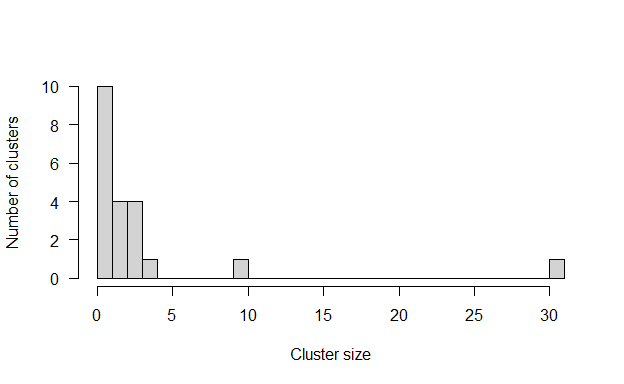Figure 2: Cluster size distribution of the simulated dataset We set up the distributions the model will use to reconstruct the transmission trees. We define f_dens as the duration of the latent period, and w_dens as the number of days between the infection dates of two connected cases, also called the serial interval. These distributions have previously been described for measles outbreaks. # Distribution of the latent period f_dens <- dgamma(x = 1:100, scale = 0.43, shape = 26.83) # Distribution of the generation time w_dens <- dnorm(x = 1:100, mean = 11.7, sd = 2.0) The age specific social contact patterns can be imported from the element age_contact of the list toy_outbreak_short. Alternatively, one can use the R package socialmixr to import a social contact matrix from the POLYMOD survey. # From the list toy_outbreak_short a_dens <- toy_outbreak_short$age_contact
# Alternatively, from POLYMOD:
polymod_matrix <-
t(socialmixr::contact_matrix(socialmixr::polymod,
countries="United Kingdom",
age.limits=seq(0, 70, by=5),
missing.contact.age="remove",
estimated.contact.age="mean",
missing.participant.age="remove")$matrix) polymod_matrix <-data.table::as.data.table(polymod_matrix) # Compute the proportion of connection to each age group a_dens <- t(t(polymod_matrix)/colSums(polymod_matrix)) Finally, the distance matrix between regions is set up from the data table dt_regions, element of toy_outbreak_short. We use the column population to set up the population vector pop_vect. We compute the distance between each region into the distance matrix dist_mat using the package geosphere. # Extract all regions in the territory dt_regions <- toy_outbreak_short[["dt_regions"]] # Extract the population vector pop_vect <- dt_regions$population
# Create the matrices of coordinates for each region (one "from"; one "to")
mat_dist_from <- matrix(c(rep(dt_regions$long, nrow(dt_regions)), rep(dt_regions$lat, nrow(dt_regions))), ncol = 2)
mat_dist_to <- matrix(c(rep(dt_regions$long, each = nrow(dt_regions)), rep(dt_regions$lat, each = nrow(dt_regions))),
ncol = 2)
# Compute all the distances between the two matrices
all_dist <- geosphere::distGeo(mat_dist_from, mat_dist_to)
# Compile into a distance matrix
dist_mat <- matrix(all_dist/1000, nrow = nrow(dt_regions))
# Rename the matrix columns and rows, and the population vector
names(pop_vect) <- rownames(dist_mat) <- colnames(dist_mat) <-
dt_regions$region Now that all the distributions and matrices are set up, we create the lists data, config, moves, likelihoods and priors to run the main function of the package. In this example, we use the default parameters to build moves, likelihoods and priors with the same elements as described in section “Implementation”. The list data contains the distributions f_dens and w_dens, the population vector and the distance matrix, along with the onset dates, age group, location and genotype of the cases. We implement two different models: in out1 the import status of the cases is inferred by the model, whereas in out2 the import status is set before the MCMC. The first short run in out1 is run with $$1,500$$ iterations to find the import status, and the main run lasts for $$5,000$$ iterations in both models. As the import status of the cases is inferred in out1, we have to set a threshold to quantify what is an unlikely likelihood of transmission between cases. We use a relative outlier threshold at 0.9, which means that the threshold will be the $$9^{th}$$ decile of the negative log-likelihoods $$L_i(t_i, j, t_j, \theta)$$ in every sample. # Default moves, likelihoods and priors moves <- custom_moves() likelihoods <- custom_likelihoods() priors <- custom_priors() # Data and config, model 1 data1 <- outbreaker_data(dates = dt_cases$Date, #Onset dates
age_group = dt_cases$age_group, #Age group region = dt_cases$Cens_tract, #Location
genotype = dt_cases$Genotype, #Genotype w_dens = w_dens, #Serial interval f_dens = f_dens, #Latent period a_dens = a_dens, #Age stratified contact matrix population = pop_vect, #Population distance = dist_mat #Distance matrix ) config1 <- create_config(data = data1, n_iter = 5000, #Iteration number: main run n_iter_import = 1500, #Iteration number: short run burnin = 500, #burnin period: first run outlier_relative = T, #Absolute / relative threshold outlier_threshold = 0.9 #Value of the threshold ) # Run model 1 out1 <- outbreaker(data = data1, config = config1, moves = moves, priors = priors, likelihoods = likelihoods) # Set data and config for model 2 data2 <- outbreaker_data(dates = dt_cases$Date,
age_group = dt_cases$age_group, region = dt_cases$Cens_tract,
genotype = dt_cases$Genotype, w_dens = w_dens, f_dens = f_dens, a_dens = a_dens, population = pop_vect, distance = dist_mat, import = dt_cases$import #Import status of the cases
)
config2 <- create_config(data = data2,
find_import = FALSE, # No inference of import status
n_iter = 5000,
sample_every = 50, # 1 in 50 iterations is kept
burnin = 500)
# Run model 2
out2 <- outbreaker(data = data2, config = config2, moves = moves,
priors = priors, likelihoods = likelihoods)

The matrices out1 and out2 now contain the posterior, likelihood, and prior of the trees generated at every iteration, along with the values of the spatial parameters a and b, the conditional report ratio pi, and the index, estimated infection date and number of generations for each case.

The function summary prints a summary of the features of the output matrix generated by outbreaker(). The function generates a list with the number of steps, the distributions of the posterior, likelihood and priors, the parameter distributions, the most likely infector and the probability of being an import for each case, and the cluster size distribution. For example, we can use it to describe the distribution of the inferred parameters. We remove the burn-in period, which corresponds to the number of iterations before the MCMC converged.

burnin <- config1$burnin # Summary parameters a and b #Model 1 print(summary(out1, burnin = burnin)$a)
##    Min. 1st Qu.  Median    Mean 3rd Qu.    Max.
##  0.2010  0.8095  0.9856  0.9846  1.2577  1.4954
print(summary(out1, burnin = burnin)$b) ## Min. 1st Qu. Median Mean 3rd Qu. Max. ## 0.08048 0.09350 0.10109 0.10170 0.10963 0.13138 # Model 2 print(summary(out2, burnin = burnin)$a)
##    Min. 1st Qu.  Median    Mean 3rd Qu.    Max.
##  0.2170  0.6942  0.8386  0.8913  1.1756  1.4892
print(summary(out2, burnin = burnin)$b) ## Min. 1st Qu. Median Mean 3rd Qu. Max. ## 0.09716 0.12132 0.12914 0.12986 0.13770 0.18719 ### Model evaluation In order to evaluate the fit of the model, we plot the median inferred cluster size distribution in out1 and out2 and the credible intervals, and compare it with the reference data. First, we group together clusters of similar sizes. The breaks of each group is written in the vector group_cluster. In this example, we defined the size categories as $$1$$; $$2$$; $$3-4$$; $$5-9$$; $$10-15$$; $$15-40$$ and $$40+$$. The inferred cluster size distributions are shown in the element cluster of the list generated by summary(out1), and can be aggregated using the input variable group_cluster. The generated barplot shows that the number of isolated cases in out1 is lower than in the data. Therefore, when the import status of cases was inferred, the model underestimated the number of clusters and tended to link together unrelated cases (Figure 3). On the other hand, the cluster size distribution in out2 is very similar to the data. Nevertheless, the cluster size distribution when the import status of the cases is inferred depends on the likelihood threshold set in outlier_threshold and outlier_relative. Using a looser or stronger definitions of $$\lambda$$ would impact the cluster size distribution inferred in out1. # We create groups of cluster size: initialise the breaks for each group group_cluster <- c(1, 2, 3, 5, 10, 15, 40, 100) - 1 # Reference data: h$counts
h <- hist(table(dt_cases$cluster), breaks = group_cluster, plot = FALSE) # Grouped cluster size distribution in each run clust_infer1 <- summary(out1, burnin = burnin, group_cluster = group_cluster)$cluster
clust_infer2 <- summary(out2, group_cluster = group_cluster,
burnin = burnin)$cluster # Merge inferred and reference cluster size distributions into one matrix clust_size_matrix <- rbind(clust_infer1["Median",], clust_infer2["Median",], h$counts)
# Plot
b <- barplot(clust_size_matrix, names.arg = colnames(clust_infer1), las=1,
ylab = "Number of clusters", xlab = "Cluster size", main = "",
beside = T, ylim = c(0, max(c(clust_infer1, clust_infer2))))
arrows(b[1,], clust_infer1["1st Qu.",], b[1,], clust_infer1["3rd Qu.",],
angle = 90, code = 3, length = 0.1)
arrows(b[2,], clust_infer2["1st Qu.",], b[2,], clust_infer2["3rd Qu.",],
angle = 90, code = 3, length = 0.1)
legend("topright", fill = grey.colors(3), bty = "n",
legend = c("Inferred import status",
"Known import status", "Simulated dataset"))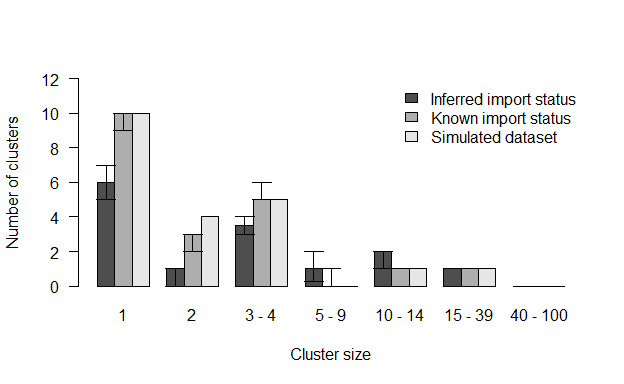Figure 3: Comparison of inferred cluster size distribution with the reference data

Although the aggregated cluster size distributions are close to the reference data, we have to investigate the reconstructed transmission trees to ensure the index assigned to each case is in agreement with the reference dataset. We write two functions: in index_infer we compute the proportion of iterations where the inferred index is the actual index for each case (perfect match); In index_clust we compute the proportion of iterations where the inferred index is from the same reference cluster as the actual index (close match).

#' Title: Compute the proportion of iterations in the outbreaker() output
# where the inferred index matches the actual index in dt_cases
#'
#' @param dt_cases: reference dataset
#' @param out: Matrix output of outbreaker()
#' @param burnin: Numeric, length of the burnin phase
#'
#' @return Numeric vector showing the proportion of iterations pointing to
#' the correct index case
index_infer <- function(dt_cases, out, burnin){
out_index <- out[out$step > burnin, grep("alpha", colnames(out))] ID_index <- matrix(dt_cases[unlist(out_index), ID], ncol = nrow(dt_cases)) match_infer_data <- t(ID_index) == dt_cases$infector_ID
match_infer_data[is.na(t(ID_index)) & is.na(dt_cases$infector_ID)] <- TRUE prop_correct <- rowSums(match_infer_data, na.rm = T)/ncol(match_infer_data) return(prop_correct) } # Same as index_infer, except it returns the proportion of inferred indexes # who are in the same reference cluster as the case index_clust <- function(dt_cases, out, burnin){ out_index <- out[out$step > burnin, grep("alpha", colnames(out))]
clust_index <- matrix(dt_cases[unlist(out_index), cluster],
ncol = nrow(dt_cases))
match_infer_data <- t(clust_index) == dt_cases$cluster match_infer_data <- match_infer_data[!is.na(dt_cases$infector_ID),]

prop_correct <- rowSums(match_infer_data, na.rm = T)/ncol(match_infer_data)

return(prop_correct)
}
# Run index_infer for each model
index_infer1 <- index_infer(dt_cases = dt_cases, out = out1, burnin = burnin)
index_infer2 <- index_infer(dt_cases = dt_cases, out = out2, burnin = burnin)
# Run index_clust for each model
index_clust1 <- index_clust(dt_cases = dt_cases, out = out1, burnin = burnin)
index_clust2 <- index_clust(dt_cases = dt_cases, out = out2, burnin = burnin)

The plots show that inferring the import status of cases decreased the proportion of perfect and close match for most cases (Figure 4). This highlights the importance of using reliable contact tracing investigations to investigate the import status of cases.

# Plot the sorted proportion in each model
par(bty = "n", mfrow = c(1, 2), mar = c(5,4,2,0), oma = c(0, 0, 0, 0))
# Panel A
plot(sort(index_infer1), type = "l", ylab = "Proportion", xlab = "Case",
main =  "A", las=1, col = grey.colors(3), lwd = 3)
lines(sort(index_infer2), col = grey.colors(3), lwd = 3)

# Panel B
plot(sort(index_clust1), type = "l", xlab = "Case", ylab = "",
main =  "B", las=1, col = grey.colors(3), lwd = 3)
lines(sort(index_clust2), col = grey.colors(3), lwd = 3)
legend("bottomright", col = grey.colors(3)[1:2], lwd = 3, bty = "n",
legend = c("Inferred import status",
"Known import status"))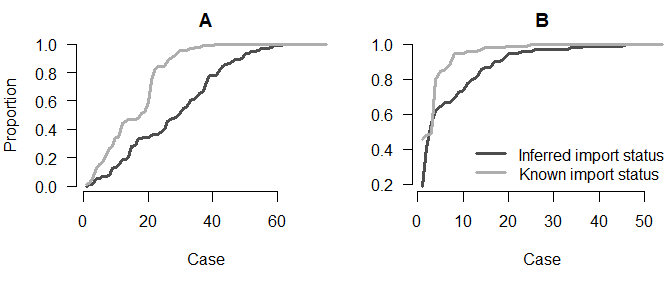Figure 4: Panel A: Proportion of iterations with the correct index for each case; Panel B: Proportion of iterations where the index is from the correct cluster

### Interpretation of the results

We now generate two maps to investigate the geographical distribution of the importations, and the average number of secondary cases per region in out1 and out2. The maps are generated using the package ggplot2

First, we retrieve the boundary files of the census tract in Ohio. They will be used to generate the background of the maps, and are available using the library tigris. The boundary files are also available on the website census.gov. We import them in a format compatible with the package sf and create one background map for each model.

library(ggplot2)
# Read the shapefile and create one map for each model
map1 <- tigris::tracts(state = "Ohio", class = "sf", progress_bar = FALSE)
map1$INTPTLON <- as.numeric(map1$INTPTLON)
map1$INTPTLAT <- as.numeric(map1$INTPTLAT)
map2 <- map1
map1$model <- "Model 1" map2$model <- "Model 2"

We are interested in two outputs of the models: i) the number of imports per region to show the regions where importations of cases are most likely, and ii) the geographical distribution of the number of secondary cases per case, which give insight into the areas most susceptible to the spread of the disease.

We compute the proportion of iterations where each case was an import in out1 and out2. The element tree of summary(out1) gives the most likely infector, the proportion of iterations where the index is the most likely infector (i.e. the support of the connection) and the median number of generations between the two cases, the most likely infection date and the chances of being an import for each case. We therefore add the columns prop_infer1 and prop_infer2 to dt_cases. As the import status is not inferred in out2, prop_infer2 is a binary value, and is the same as dt_cases$import. # Add the proportion of iteration in model 1 where each case is an import dt_cases[, prop_infer1 := summary(out1, burnin = burnin)$tree$import] # Add the proportion of iteration in model 2 where each case is an import dt_cases[, prop_infer2 := summary(out2, burnin = burnin)$tree$import] We then aggregate the number of imports per region in each model, and name these vectors prop_reg1 and prop_reg2. We then add the number of imports in each region to the matrices describing the maps. # Number of import per region in model 1 prop_reg1 <- dt_cases[, .(prop_per_reg = sum(prop_infer1)), by = Cens_tract]$prop_per_reg
# Number of import per region in model 2
prop_reg2 <- dt_cases[, .(prop_per_reg = sum(prop_infer2)),
by = Cens_tract]$prop_per_reg names(prop_reg1) <- names(prop_reg2) <- unique(dt_cases$Cens_tract)

# Add the number of imports in each region to the maps
map1$prop_reg <- prop_reg1[as.character(map1$GEOID)]
map2$prop_reg <- prop_reg2[as.character(map2$GEOID)]

We now plot the number of imports per region in each model (Figure 5). Regions where no cases were reported are shown in grey. The right panel (Model out2) shows the geographical distribution of importations in the data.

We observe discrepancies between the two panels: although some regions have the correct number of imports inferred in most iterations, there are uncertainties for some imports in the left panel. Furthermore, the right panel shows there should be one import in 4 of the regions in the central area, which seems to be underestimated in the left panel. These maps highlight the uncertainty added by the inference of the import status of each case.

# Merge maps
maps <- rbind(map1, map2)

limits_long <- c(-84, -82)
limits_lat <- c(40, 41.5)
maps <- maps[maps$INTPTLON > limits_long & maps$INTPTLON < limits_long,]
maps <- maps[maps$INTPTLAT > limits_lat & maps$INTPTLAT < limits_lat,]

# Plot: number of imports per region, two panels
ggplot(maps) +  geom_sf(aes(fill = prop_reg)) + facet_grid(~model)  +
scale_fill_gradient2(na.value = "lightgrey", midpoint = 0.8,
breaks = c(0, 0.5, 1, 1.5), name = "Nb imports",
low = "white", mid = "lightblue", high = "darkblue") +
coord_sf(xlim = c(-83.8, -82.2), ylim = c(40.2, 41.3)) +
theme_classic(base_size = 9)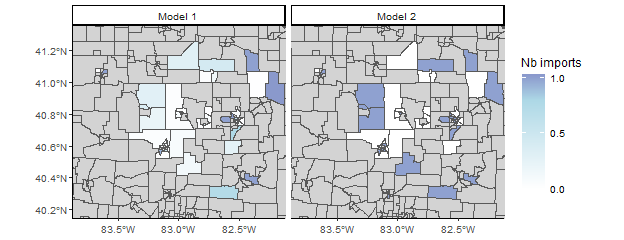Figure 5: Average number of import cases per census tract

We now compute the average number of secondary cases per case in each region. We define the function n_sec_per_reg¬, which computes the number of secondary cases per case and aggregates it per region at each iteration of the model. We then extract the median number of secondary cases per case in each region. The dispersion of the number of secondary cases per region can be generated by taking another quantile than the median. For example,n_sec1 <- apply(n_sec_tot1[,-1], 1, function(X) quantile(X, 0.25)) would show the first quartile in each region.

#' Title: Compute the number of secondary cases per case in each region
#'
#' @param dt_cases: reference dataset
#' @param out: Matrix output of outbreaker()
#' @param burnin: Numeric, length of the burnin phase
#'
#' @return A numeric matrix: the first column is the census tract ID, the
#' other columns show the number of secondary cases per case. Each row
#' corresponds to a different iteration.
n_sec_per_reg <- function(dt_cases, out, burnin){
## Number of secondary cases per case
n_sec <- apply(out[out$step > burnin, grep("alpha", colnames(out))], 1, function(X){ X <- factor(X, 1:length(X)) return(table(X))}) ## Aggregate by region tot_n_sec_reg <- aggregate(n_sec, list(dt_cases$Cens_tract), sum)
## Divide by the number of cases in each region
tot_n_sec_reg <- cbind(tot_n_sec_reg[, 1],
tot_n_sec_reg[, -1] / table(dt_cases$Cens_tract)) return(tot_n_sec_reg) } n_sec_tot1 <- n_sec_per_reg(dt_cases = dt_cases, out = out1, burnin = burnin) n_sec_tot2 <- n_sec_per_reg(dt_cases = dt_cases, out = out2, burnin = burnin) n_sec1 <- apply(n_sec_tot1[,-1], 1, median) n_sec2 <- apply(n_sec_tot2[,-1], 1, median) names(n_sec1) <- names(n_sec2) <- unique(dt_cases$Cens_tract)

We then add the number of secondary cases per region to the matrices describing the maps.

map1$n_sec <- as.numeric(n_sec1[as.character(map1$GEOID)])
map2$n_sec <- as.numeric(n_sec2[as.character(map2$GEOID)])

We can now plot the geographical distribution of the median number of secondary cases in each region (Figure 6). The maps generated by the two models are very similar. Both of them show the spatial heterogeneity of the number of secondary infections. Some regions seem to consistently cause more secondary cases. There are minor discrepancies between the maps, but they show the same pattern. If we change the vectors n_sec1 and n_sec2 to plot different deciles, we can get an idea of the dispersion of the number of secondary cases in the different iterations of the models.

# Merge maps
maps <- rbind(map1, map2)

limits_long <- c(-84, -82)
limits_lat <- c(40, 41.5)
maps <- maps[maps$INTPTLON > limits_long & maps$INTPTLON < limits_long,]
maps <- maps[maps$INTPTLAT > limits_lat & maps$INTPTLAT < limits_lat,]

# Plot the geographical distribution of the number of secondary cases
ggplot(maps) +  geom_sf(aes(fill = n_sec)) + facet_grid(~model)  +
scale_fill_gradient2(na.value = "lightgrey", mid = "lightblue",
low = "white", midpoint = 1, high = "darkblue",
breaks = seq(0, 5, 0.5),name = "Sec cases") +
coord_sf(xlim = c(-83.8, -82.2), ylim = c(40.2, 41.3)) +
theme_classic(base_size = 9)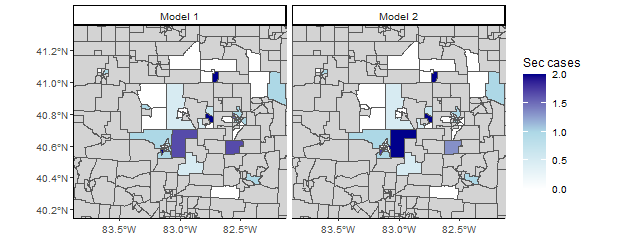Figure 6: Median number of secondary transmission per case in each census tract

## Part two: Modify the likelihoods, priors and movements lists: the Stouffer’s rank module

In the previous section, we ran and evaluated two different models to reconstruct transmission clusters from simulated surveillance data, and highlighted the spatial heterogeneity of measles transmission in the region. These models were run using the default likelihood, prior and movement functions. Now we develop a third model, where the spatial connection between regions is based on the Stouffer’s rank method.

The Stouffer’s rank model does not take absolute distance into account to compute the probability of connection between regions. In this model, the connectivity between the regions $$k$$ and $$l$$ only depends on the summed population of all the towns closer to $$l$$ than $$k$$. If we define this collection of towns $$\Omega_{k,l} = \{i: 0 \leq d(i,l) \leq d(k,l) \}$$, the distance of Stouffer is then $$p_{kl} = m_l^c * \left(\frac{m_k}{\sum_{i \in \Omega_{k,l}} m_i}\right)^a$$. From this, we deduce the probability that a case from region $$l$$ was infected by a case from region $$k$$ as $s(k,l)= \frac{p_{kl}}{\Sigma_hp_{hl}}=\frac{\left(\frac{m_k}{\sum_{i \in \Omega_{k,l}} m_i}\right)^a}{\Sigma_h\left(\frac{m_h}{\sum_{i \in \Omega_{h,l}} m_i}\right)^a}$

This model is similar to the power-law gravity model with two main differences:

• Each cell of the distance matrix should be equal to $$\sum_{i \in \Omega_{k,l}} m_i$$.

• There is only one spatial parameter $$a$$ to estimate.

First, we create the initial distance matrix in the Stouffer’s rank model dist_mat_stouffer:

# For every column of the distance matrix, use the cumulative sum of the
# population vector ordered by the distance. Remove the values where
# the distance between the regions is above gamma
dist_mat_stouffer <- apply(dist_mat, 2, function(X){
pop_X <- cumsum(pop_vect[order(X)])
omega_X <- pop_X[names(X)]
# omega_X is set to -1 if the distance between two regions is above gamma
omega_X[X > config1$gamma] <- -1 return(omega_X) }) # The new value of gamma is equal to the maximum of dist_mat_stouffer + 1 gamma <- max(dist_mat_stouffer) + 1 # The values previously set to -1 are now set to the new value of gamma dist_mat_stouffer[dist_mat_stouffer == -1] <- max(dist_mat_stouffer) * 2 We write the function cpp_stouffer_move_a to replace the default movement function cpp_move_a. As the formula used to compute the Stouffer’s rank values is similar to the power law gravity models, we do not need to re-write cpp_log_like, the default function to compute the probability matrix. Other distance models may require a rewriting of cpp_log_like, which should then be called in the new movement function. In the Stouffer’s rank model, there is no parameter $$b$$, both spatial parameters are equal to $$a$$. We use the package Rcpp to source the new movement function. // [[Rcpp::depends(o2geosocial)]] #include <Rcpp.h> #include <Rmath.h> #include <o2geosocial.h> // [[Rcpp::export()]] Rcpp::List cpp_stouffer_move_a(Rcpp::List param, Rcpp::List data, Rcpp::List config, Rcpp::RObject custom_ll, Rcpp::RObject custom_prior) { // Import parameters Rcpp::List new_param = clone(param); double gamma = config["gamma"]; int max_kappa = config["max_kappa"]; Rcpp::String spatial = config["spatial_method"]; Rcpp::IntegerVector region = data["region"]; Rcpp::NumericMatrix distance = data["distance"]; Rcpp::NumericMatrix can_be_ances_reg = data["can_be_ances_reg"]; Rcpp::NumericVector population = data["population"]; Rcpp::NumericVector limits = config["prior_a"]; // Size of the probability matrix Rcpp::List new_log_s_dens = new_param["log_s_dens"]; Rcpp::NumericMatrix probs = new_log_s_dens; int nb_cases = pow(probs.size(), 0.5); // Draw new value of a Rcpp::NumericVector new_a = new_param["a"]; double sd_a = static_cast<double>(config["sd_a"]); double old_logpost = 0.0, new_logpost = 0.0, p_accept = 0.0; // proposal (normal distribution with SD: config$sd_a)
new_a += R::rnorm(0.0, sd_a); // new proposed value
if (new_a < limits || new_a > limits) {
return param;
}
// Generate new probability matrix
new_param["log_s_dens"] = o2geosocial::cpp_log_like(population, distance,
can_be_ances_reg,
new_a, new_a,
max_kappa, gamma,
spatial, nb_cases);
// Compare old and new likelihood values
old_logpost = o2geosocial::cpp_ll_space(data, config, param,
R_NilValue, custom_ll);
new_logpost = o2geosocial::cpp_ll_space(data, config, new_param,
R_NilValue, custom_ll);
old_logpost += o2geosocial::cpp_prior_a(param, config, custom_prior);
new_logpost += o2geosocial::cpp_prior_a(new_param, config, custom_prior);
// Accept or reject proposal
p_accept = exp(new_logpost - old_logpost);
if (p_accept < unif_rand()) {
return param;
}
return new_param;
}

We modify the element $$a$$ of the list moves, and replace it with cpp_stouffer_move_a. As b is not estimated in this model, we create the null function f_null, and modify the list priors.

moves3 <- custom_moves(a = cpp_stouffer_move_a)

f_null <- function(param) {
return(0.0)
}
priors3 <- custom_priors(b = f_null)

Finally, we set up the lists data and config: the distance matrix for this run is dist_mat_stouffer; we do not move the parameter b, and we change the value of gamma. We then run out_stouffer.

# Set data and config lists
data3 <- outbreaker_data(dates = dt_cases$Date, #Onset dates age_group = dt_cases$age_group, #Age group
region = dt_cases$Cens_tract, #Location genotype = dt_cases$Genotype, #Genotype
w_dens = w_dens, #Serial interval
f_dens = f_dens, #Latent period
a_dens = a_dens, #Age stratified contact matrix
population = pop_vect, #Population
distance = dist_mat_stouffer #Distance matrix
)
config3 <- create_config(data = data3,
gamma = gamma,
move_b = FALSE, # b is not estimated
init_b = 0,
spatial_method = "power-law",
n_iter = 5000, #Iteration number: main run
n_iter_import = 1500, #Iteration number: short run
burnin = 500, #burnin period: first run
outlier_relative = T, #Absolute / relative threshold
outlier_threshold = 0.9 #Value of the threshold
)
# Run the model using the Stouffer's rank method
out_stouffer <- outbreaker(data = data3, config = config3, moves = moves3,
priors = priors3, likelihoods = likelihoods)

As we did in the previous section, we plot the inferred cluster size distribution and compare it to the reference data (Figure 7). We observe discrepancies between the inferred distribution and the data: the model over-estimates the number of larger clusters (above 5 cases).

# Grouped cluster size distribution in the Stouffer's rank model
clust_infer_stouf <- summary(out_stouffer, burnin = burnin,
group_cluster = group_cluster)$cluster # Merge inferred and reference cluster size distributions clust_size_matrix <- rbind(clust_infer_stouf["Median",], h$counts)
# Plot the two distributions
b <- barplot(clust_size_matrix, names.arg = colnames(clust_infer_stouf),
beside = T)
arrows(b[1,], clust_infer_stouf["1st Qu.",], b[1,],
clust_infer_stouf["3rd Qu.",], angle = 90, code = 3, length = 0.1)
legend("topright", fill = grey.colors(2), bty = "n",
legend = c("Inferred import status, Stouffer's rank method",
"Simulated dataset"))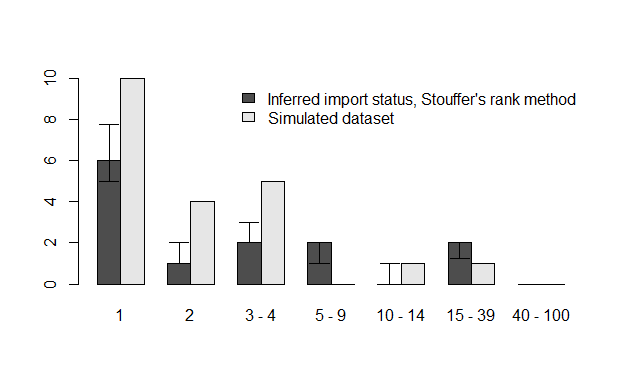Figure 7: Comparison of inferred cluster size distribution with the reference data

Finally, we plot the proportion of perfect and close match for each case (Figure 8). We observe that the fit obtained with the Stouffer’s rank method is consistently worse than the first two models. The Stouffer’s rank method did not improve the agreement between the inferred trees and the reference data.

The reference data used in the study were simulated using an exponential gravity model, which explains why introducing the Stouffer’s rank method did not improve the inference. This is not representative of the performance of each mobility model on real-world data.

index_infer_stouf <- index_infer(dt_cases = dt_cases, out = out_stouffer,
burnin = config1$burnin) index_clust_stouf <- index_clust(dt_cases = dt_cases, out = out_stouffer, burnin = config1$burnin)
# Plot the sorted proportion in each model
par(bty = "n", mfrow = c(1, 2), mar = c(5,4,2,0), oma = c(0, 0, 0, 0))
# Panel A
plot(sort(index_infer_stouf), type = "l", xlab = "Case", ylab = "",
main =  "A", las=1, col = grey.colors(2), lwd = 3)
# Panel B
plot(sort(index_clust_stouf), type = "l", ylab = "Proportion", xlab = "Case",
main =  "B", las=1, col = grey.colors(2), lwd = 3)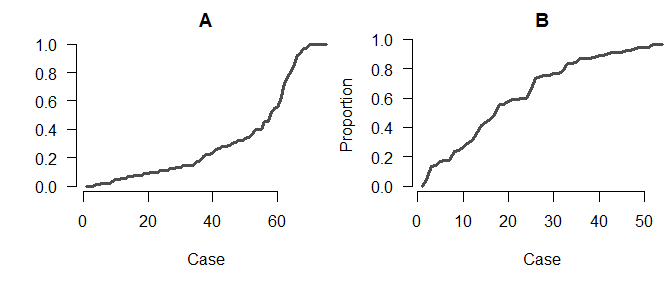Figure 8: Panel A: Proportion of iterations with the correct index for each case; Panel B: Proportion of iterations where the index is from the correct cluster

par(oldpar)

# Conclusion

The R package o2geosocial is a new tool for data analysis building upon the framework developed in outbreaker2. It is highly flexible and only requires routinely collected surveillance data. It can be used to identify large transmission clusters and highlight the dynamics of transmission in different regions. An application of the algorithm on a small geographical scale was presented in the tutorial, it can also be used to study datasets of cases scattered across larger areas. The methods presented in the tutorial can be applied to the second dataset included in the package toy_outbreak_long`, which includes more than 1900 cases simulated in the United States. The computation time increases with the number of regions and the number of cases.

We showed how the model could be edited to implement a range of mobility models. Describing human mobility during infectious diseases outbreaks can be challenging, and the performances of the models depend on the setting studied. The package can be extended to take into account the variety of existing mobility models. We encourage the development of extensions and the use of o2geosocial to study different pathogens and settings where sequence data cannot be used to reconstruct transmission clusters. We hope that wider use of o2geosocial can help maximise what can be reconstructed from routinely collected data and epidemiological investigations to improve our understanding of outbreak dynamics.